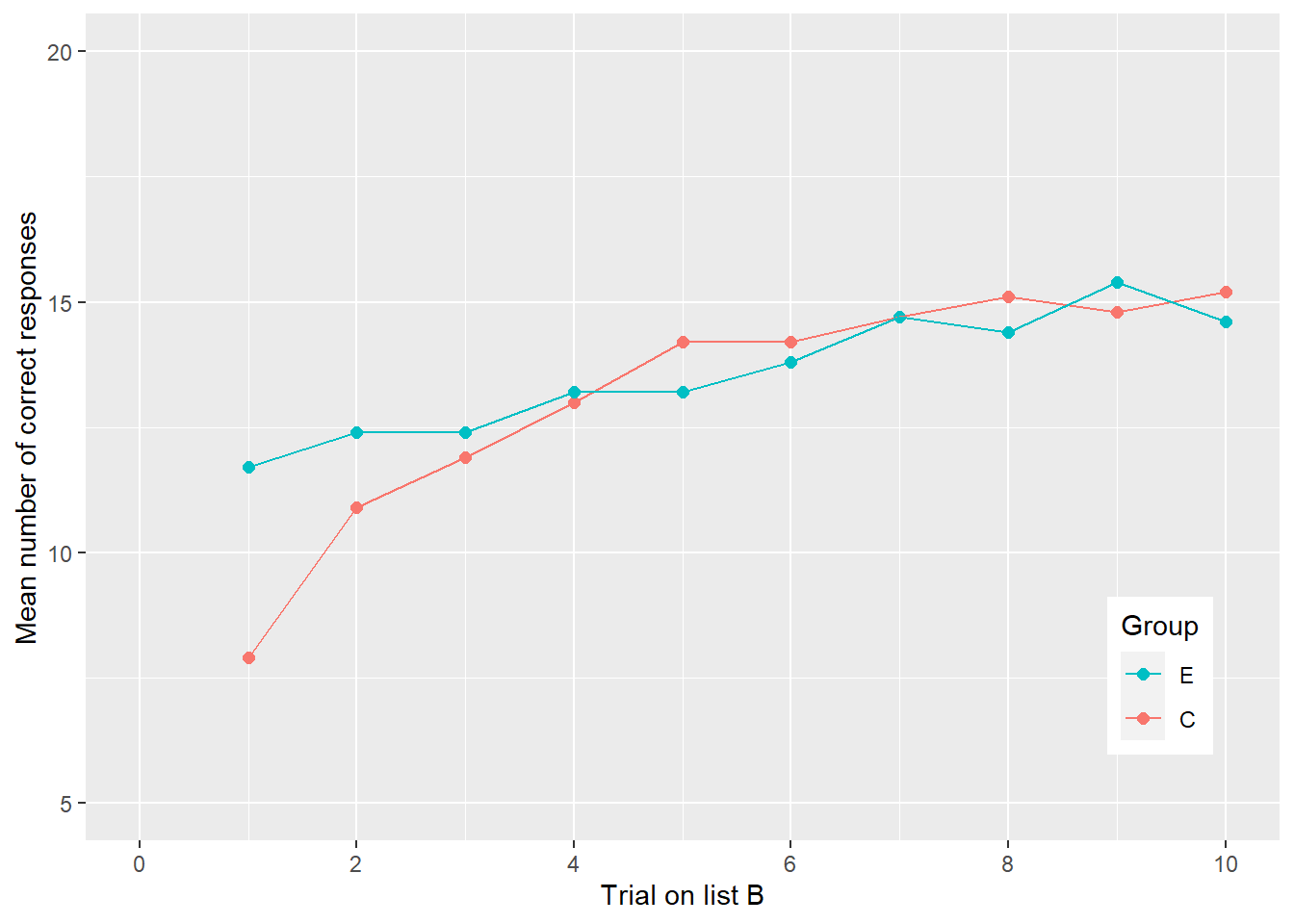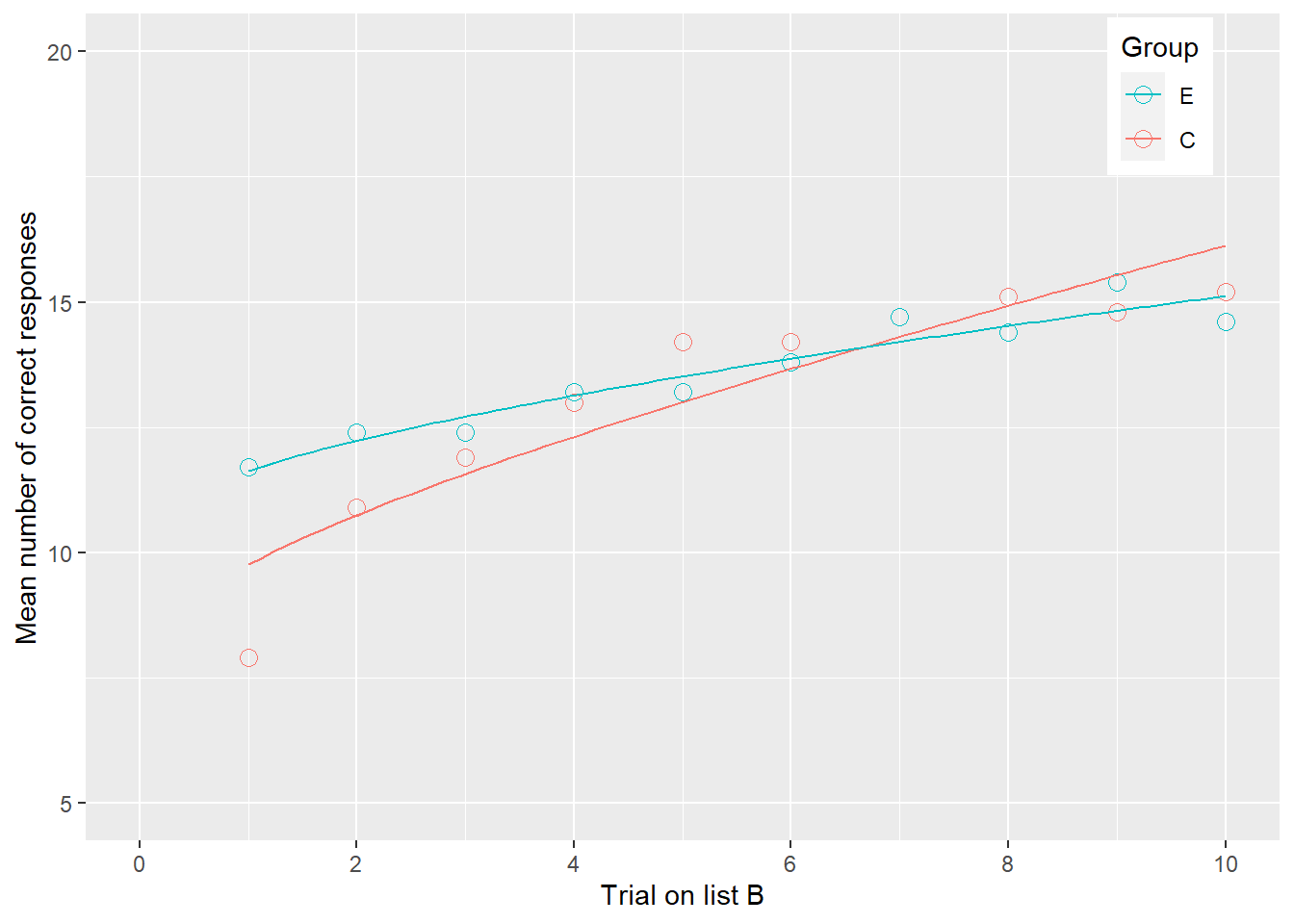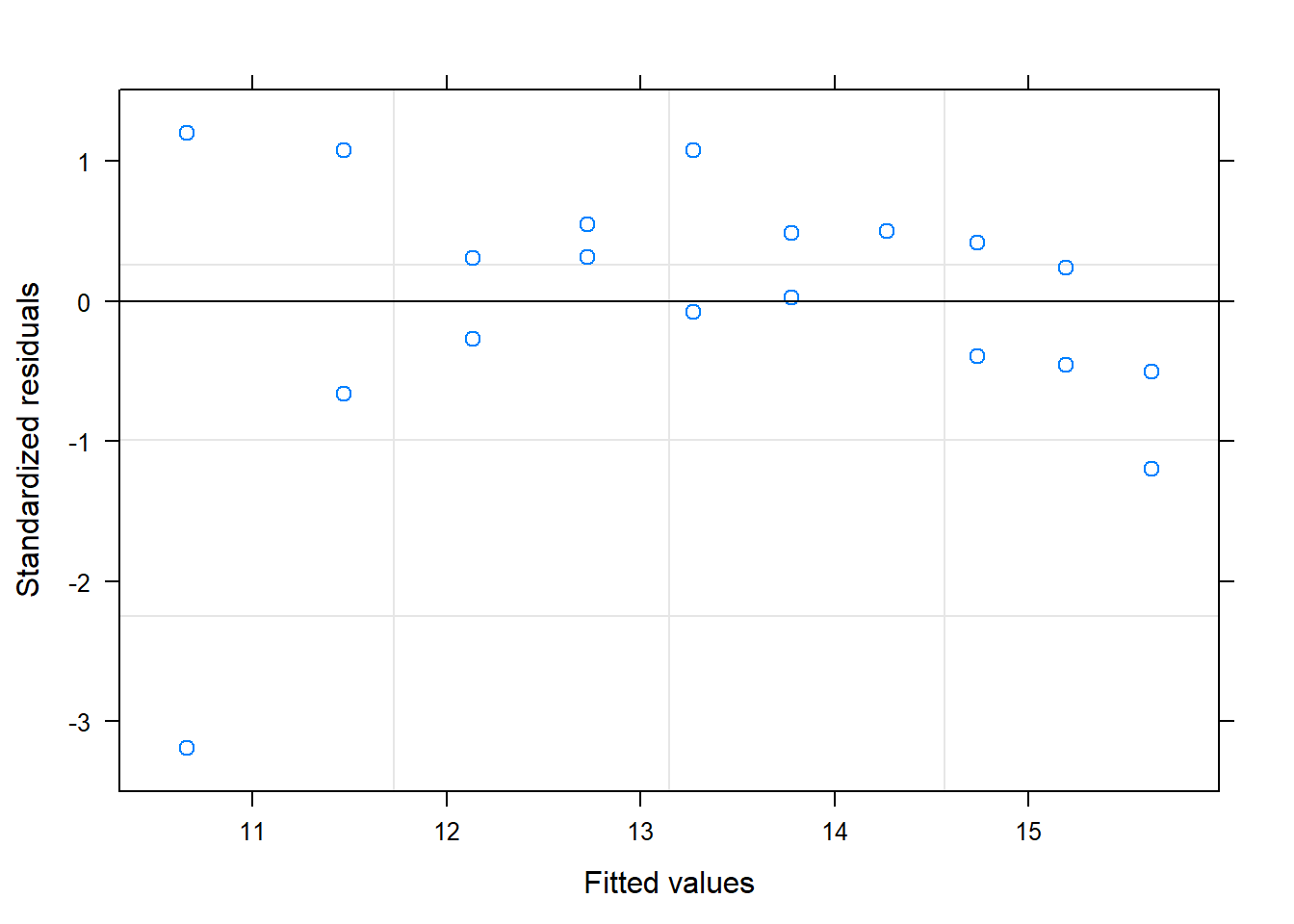# 1 Introduction

In a learning task known as a free-recall list, each subject was presented with a list of words, one at a time, for a few second each. After all the words had been presented, the subject was asked to recall as many of the words as possible. On each successive study trial the order of presenting the words differ.

Control: 2 trials on List A, followed by 10 trials on List B. Experimental: 2 trials on List B, followed by 10 trials on List B.

The mean number of correct responses given on each trial for the two group was the outcome variable.

Source: Schwartz, R.M., & Humphreys, M.S. (1973). List differentiation in part/whole free recall. American Journal of Psychology, 86, 79-88.

Data

Column 1: Time in half years unit Column 2: Percent of details recalled

# 2 Data Management

# load packages
pacman::p_load(tidyverse, nlme, car,lattice,nlstools)
# input data
dta<- read.table("C:/Users/Ching-Fang Wu/Documents/data/freeRecall.asc.txt", header = T)
# 重新命名
names(dta) <- c("Group","Trial","CorrectN")
str(dta)
## 'data.frame':    20 obs. of  3 variables:
##  $Group : chr "C" "C" "C" "C" ... ##$ Trial   : int  1 2 3 4 5 6 7 8 9 10 ...
##  $CorrectN: num 7.9 10.9 11.9 13 14.2 14.2 14.7 15.1 14.8 15.2 ... # 3 Plot ggplot(dta, aes(Trial, CorrectN, color = Group)) + geom_point(size = rel(2)) + geom_line() + labs(x = "Trial on list B", y = "Mean number of correct responses")+ scale_x_continuous(limits = c(0, 10), breaks = seq(0, 10, by = 2)) + scale_y_continuous(limits = c(5, 20), breaks = seq(5, 20, by = 5)) + guides(color = guide_legend(reverse = T)) + theme(legend.position = c(.9,.2))# 4 model Model: CRi = α × exp(β sqrt(triali)) initial <- c(a = 5, b = log(10/5)) summary(m0h <- nls(CorrectN ~ a*exp(b*sqrt(Trial)), data = dta, start = initial)) ## ## Formula: CorrectN ~ a * exp(b * sqrt(Trial)) ## ## Parameters: ## Estimate Std. Error t value Pr(>|t|) ## a 8.92939 0.50253 17.769 7.34e-13 *** ## b 0.17714 0.02269 7.806 3.47e-07 *** ## --- ## Signif. codes: 0 '***' 0.001 '**' 0.01 '*' 0.05 '.' 0.1 ' ' 1 ## ## Residual standard error: 0.864 on 18 degrees of freedom ## ## Number of iterations to convergence: 6 ## Achieved convergence tolerance: 4.602e-07 # 5 dta$yhat1 <- fitted(m0h)

ggplot(dta, aes(Trial, CorrectN, color = Group)) +
geom_point(pch = 1, size = 3) +
geom_smooth(method = "nls", formula = y ~ a*exp(b*sqrt(x)),
method.args = list(start = initial),
se = F,
size = rel(.5))+
scale_x_continuous(limits = c(0, 10), breaks = seq(0, 10, by = 2)) +
scale_y_continuous(limits = c(5, 20), breaks = seq(5, 20, by = 5)) +
guides(color = guide_legend(reverse = T)) +
labs(x = "Trial on list B", y = "Mean number of correct responses")+
theme(legend.position = c(.9,.9))# 6 residual plot

plot(m0h)#The end